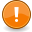RandIntThis function is deprecated. This means that its use is discouraged and that it might not exist in future versions. Please use math.random instead.

This function returns a random integer value between a specified minimum and maximum value.

Syntax

int randInt ( int lowerbound, int upperbound )

Required Arguments

• lowerbound: This is the lowest random value this function can return.
• upperbound: This is the highest random value this function can return.

Returns

Returns an int containing a random number between and potentially including lowerbound and upperbound.

Example

This example outputs a random number between 5 and 10 inclusive.

outputChatBox ( "A random value between 5 and 10 is " .. randInt ( 5, 10 ) )